#### Chapter 8 Lines and Angle R.D. Sharma Solutions for Class 9th Math MCQ

Multiple Choice Questions

1. One angle is equal to three times its supplement. The measure of the angle is
(a) 130°
(b) 135°
(c) 90°
(d) 120°

Solution2. Two complementary angles are such that two times the measure of one is equal to three times the measure of the other. The measure of the smaller angle is
(a) 45°
(b) 30°
(c) 36°
(d) none of these

Solution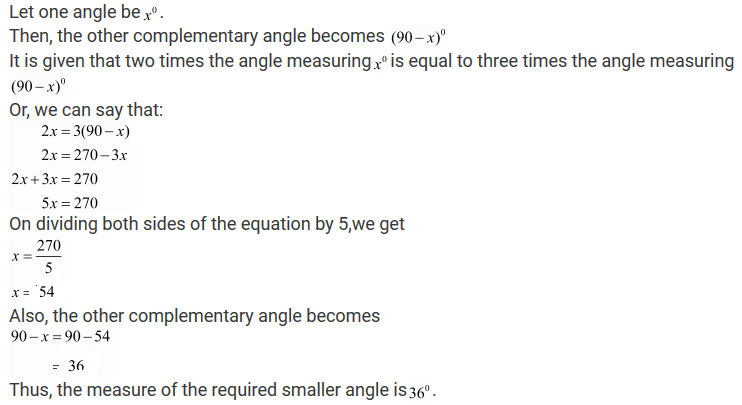3. Two straight line AB and CD intersect one another at the point O. If ∠AOC + ∠COB + ∠BOD = 274°, then ∠AOD =
(i) 86°
(ii) 90°
(iii) 94°
(iv) 137°

Solution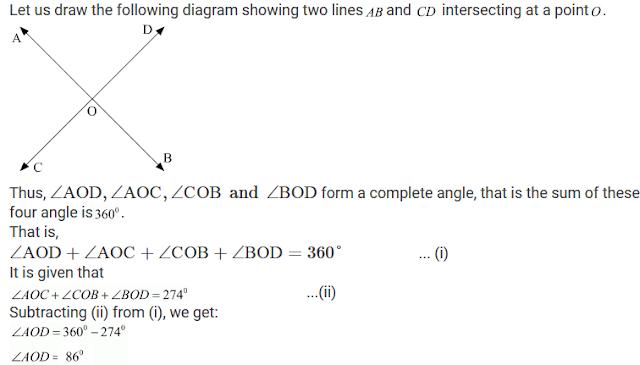4. Two straight lines AB and CD cut each other at O. If ∠BOD = 63°, then ∠BOC =
(a) 63°
(b) 117°
(c) 17°
(d) 153°

Solution5. Consider the following statements:
When two straight lines intersect:

(iii) opposite angles are equal
(iv) opposite angles are supplementary

Of these statements

(a) (i) and (ii) are correct
(b) (ii) and (iii) are correct
(c) (i) and (iv) are correct
(d) (ii) and (iv)  are correct

Solution

Let us draw the following diagram showing two straight lines AD and BC intersecting each other at a point O.Now, let us consider each statement one by one:

(i) When two lines intersect adjacent angles are complementary.
This statement is incorrect
Explanation:
As the adjacent angles form a linear pair and they are supplementary.

(ii) When two lines intersect adjacent angles are supplementary.
This statement is correct.
Explanation:
As the adjacent angles form a linear pair and they are supplementary.
(iii) When two lines intersect opposite angles are equal.
This statement is correct.
Explanation:
As the vertically opposite angles are equal.

(iv) When two lines intersect opposite angles are supplementary.
This statement is incorrect.
Explanation:
As the vertically opposite angles are equal
Thus, out of all, (ii) and (iii) are correct.
Hence, the correct choice is (b).

6. Given ∠POR = 3x and ∠QOR = 2x + 10°. If POQ is a straight line, then the value of x is
(a) 30°
(b) 34°
(c) 36°
(d) none of these

Solution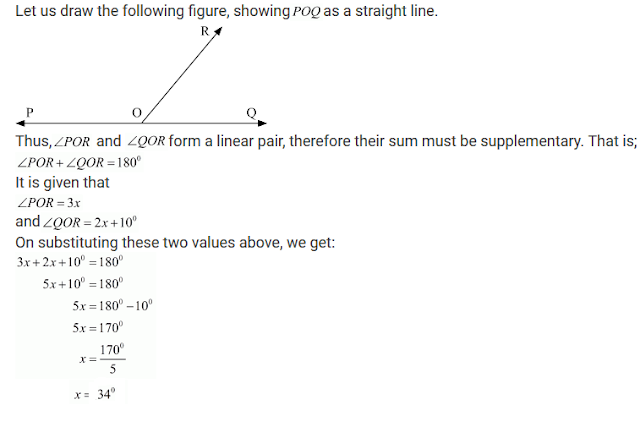7. In Fig. 8.122, AOB is a straight line. If ∠AOC + ∠BOD = 85°, then ∠COD =
(a) 85°
(b) 90°
(c) 95°
(d) 100°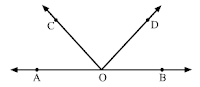Solution8. In Fig. 8.123, the value of y is
(a) 20°
(b) 30°
(c) 45°
(d) 60°Solution9. In Fig. 8.124, if y/x = 5 and z/x = 4, then the value of x is
(a) 8
(b) 18
(c) 12
(d) 15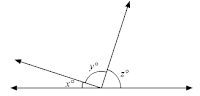Solution10. In Fig. 8.125, the value of x is
(a) 12
(b) 15
(c) 20
(d) 30Solution11. In Fig. 8.126, which of the following statements must be true?
(i) a + b = d + c
(ii) a + c + e = 180°
(iii) b + f = c + e

(a) (i) only

(b) (ii) only
(c) (iii) only
(d) (ii) and (iii) onlySolution

(i) Statement: a+b = d+c
This statement is incorrect.
Explanation: We have, a and dare vertically opposite angles.
Therefore, a=d ---(i)
Similarly, band e are vertically opposite angles.
Therefore,
b=e ---(ii)
On adding (i) and (ii), we get
a+b=d+e
Thus, this statement is incorrect.

(ii) Statement a+c+e = 180°
This statement is correct.
Explanation: As a°, f° and e° form a linear pair, therefore their sum must be supplementary.
a+f+e = 180° ---(iii)
Also f° and c° are vertically opposite angles, therefore, these must be equal.
f =c
Putting f=c in (iii), we get:
a+c+e=180°

(iii) Statement: b+ f =a+e
This statement is correct.
Explanation:  As a°, f° and b° form a linear pair, therefore their sum must be supplementary.
a+ f +b=180° ---(iv)
Also c°,d° and e° form a linear pair, therefore their sum must be supplementary.
c+d+e=180° ---(v)
On comparing (iv) and (v), we get:
a+ f +b=c+d+e
Also a° and d° are vertically opposite angles, therefore, these must be equal.
Therefore,
a= d
Substituting the above equation in (vi), we get:
a+f+b = c+d+e
a+b+f = c+a+e
b+f = a+e
Thus, out of all, (ii) and (iii) are correct.

12. If two interior angles on the same side of a transversal intersecting two parallel lines are in the ratio 2:3, then the measure of the larger angle is
(a) 54°
(b) 120°
(c) 108°
(d) 136°

Solution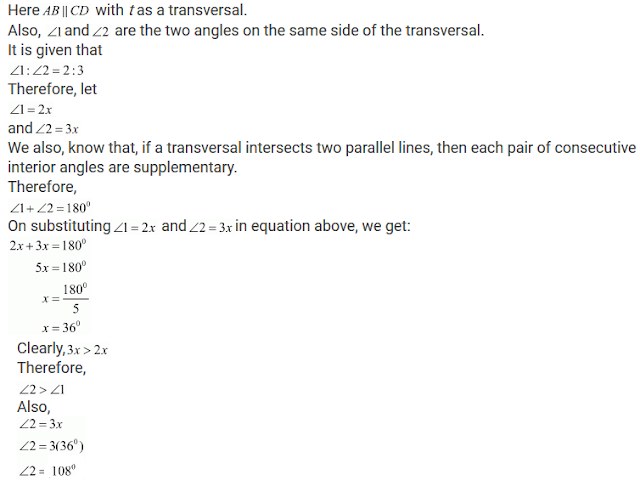13. In Fig. 8.127, AB || CD || EF and GH || KL. The measure of ∠HKL is
(a) 85°
(b) 135°
(c) 145°
(d) 215°Solution14. In Fig. 8.128, if AB || CD, then the value of x is
(a) 20°
(b) 30°
(c) 45°
(d) 60°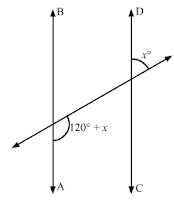Solution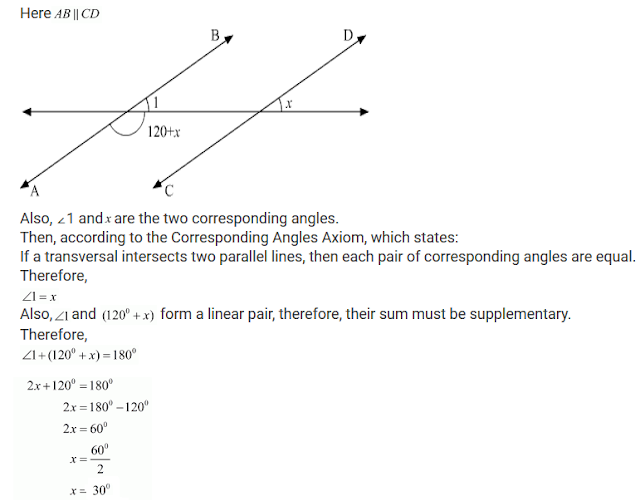15. AB and CD are two parallel lines. PQ cuts AB and CD at E and F respectively. EL is the bisector of ∠FEB. If ∠LEB = 35°, then ∠CFQ will be

(a) 55°
(b) 70°
(c) 110°
(d) 130°

Solution16. Two lines AB and CD intersect at O. If ∠AOC + ∠COB + ∠BOD = 270°, then ∠AOC =

(a) 70°
(b) 80°
(c) 90°
(d) 180°

Solution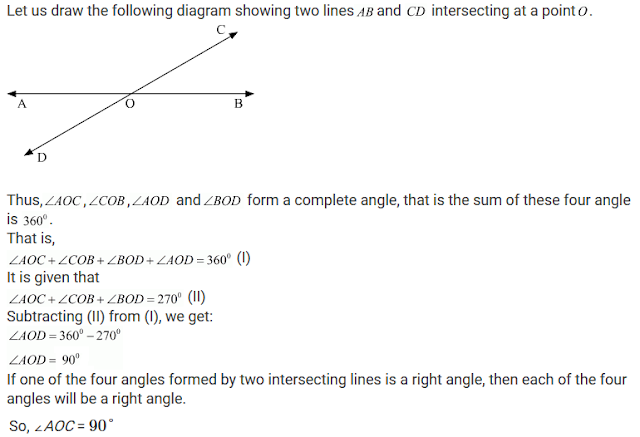17. In Fig. 8.129, PQ || RS, ∠AEF = 95°, ∠BHS = 110° and ∠ABC = x°. Then the value of x is

(a) 15°
(b) 25°
(c) 70°
(d) 35°SolutionSolution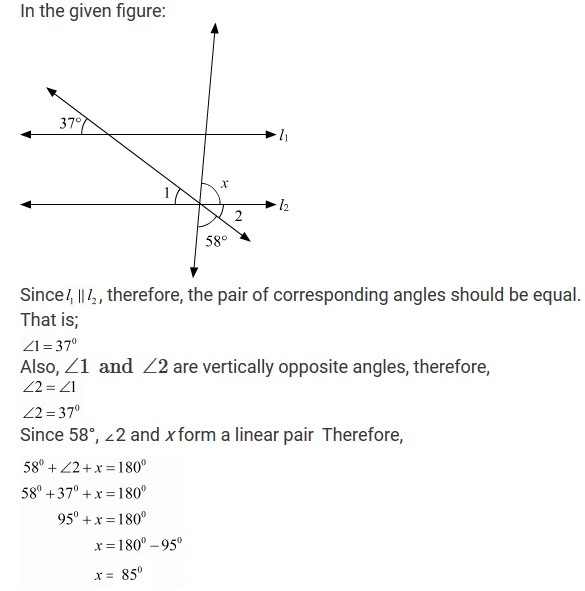19. In Fig. 8.131, if l1∥l2, what is x+y in terms of w and z?
(a) 180-w+z
(b) 180+w-z
(c) 180-w-z
(d) 180+w+z

Solution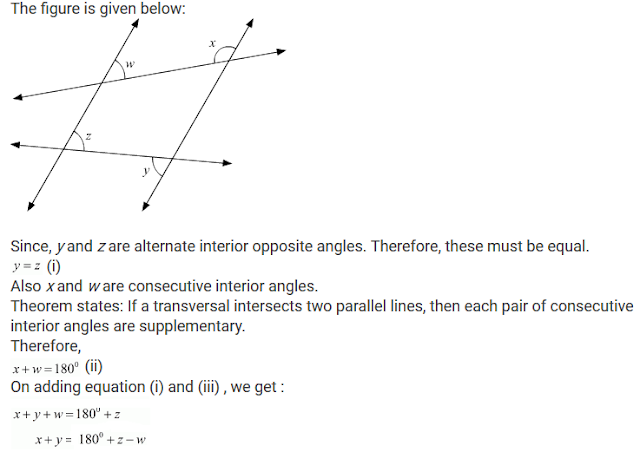20. In Fig. 8.132, if l1∥l2, what is the value of y?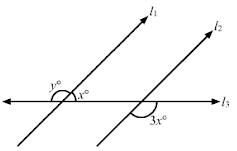(a) 100
(b) 120
(c) 135
(d) 150

Solution21. In Fig. 8.133, if l1∥l2 and l3∥l4, what is y in terms of x?
(a) 90+x
(b) 90+2x
(c) 90 - x/2
(d) 90-2x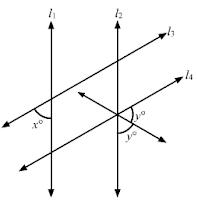Solution22. In Fig. 8.13, if l∥m, what is the value of x?(a) 60
(b) 50
(c) 45
(d) 30

Solution23. In Fig. 8.135, if the line segment AB is parallel to the line segment CD, what is the value of y?
(a) 12
(b) 15
(c) 18
(d) 20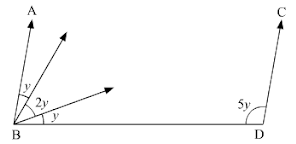Solution24. In Fig. 8.136, if CP∥DQ, then the measure of x is
(a) 130°
(b) 105°
(c) 175°
(d) 125°Solution25. In Fig. 8.137, if AB || HF and DE || FG, then the measure of ∠FDE is
(a) 108°
(b) 80°
(c) 100°
(d) 90°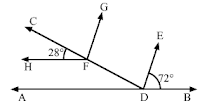Solution26. In Fig. 8.138, if lines l and m are parallel then x =
(a) 20°
(b) 45°
(c) 65°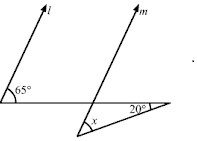Solution27. In Fig. 8.139, if AB∥CD, then x =
(a) 100°
(b) 105°
(c) 110°
(d) 115°Solution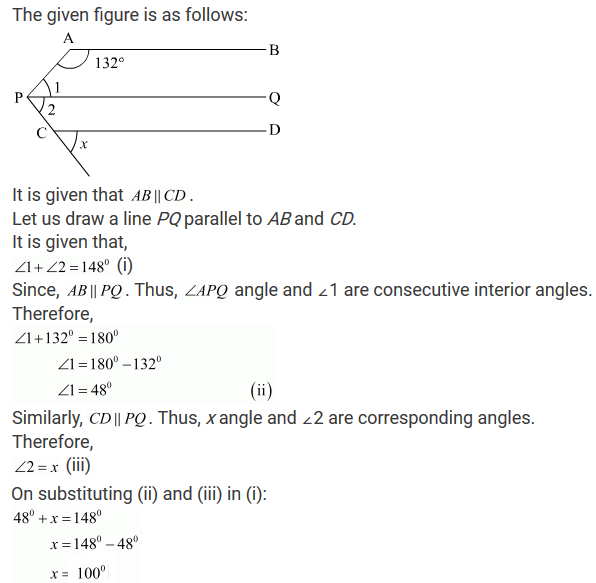28. In Fig. 8.140, if lines l and m are parallel lines, then x =
(a) 70°
(b) 100°
(c) 40°
(d) 30°Solution29. In Fig. 8.141, if l∥m, then x =
(a) 105°
(b) 65°
(c) 40°
(d) 25°Solution30. In Fig. 8.142, if lines l and m are parallel, then the value of x is
(a) 35°
(b) 55°
(c) 65°
(d) 75°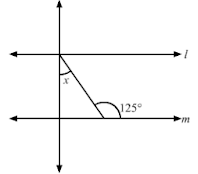Solution